# Colleges with the lowest SAT scores in Arkansas

Top 10 colleges in Arkansas with the lowest SAT scores
Looking for the colleges with the lowest SAT scores in Arkansas? Well you're in luck! We've compiled a national college database and have created a list of the top 10 universities with the lowest SAT scores in Arkansas below. If you are not a good test taker or worried about your test scores, this list is for you. These are the schools whose applicants had the lowest average SAT scores in Arkansas, which means that you can get into these colleges with a lower SAT score. We also include each college's ACT scores and acceptance rate so that you can see where you would have the easiest time getting in. Read on to find out more.

## University of Arkansas at Pine Bluff SAT scores

The average SAT score for University of Arkansas at Pine Bluff is 980.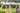The average SAT score of 980 breaks down into:

• SAT math: 480

The average ACT score for University of Arkansas at Pine Bluff is 18 and their acceptance rate is 46.7%.

## Central Baptist College SAT scores

The average SAT score for Central Baptist College is 990.The average SAT score of 990 breaks down into:

• SAT math: 470

The average ACT score for Central Baptist College is 21 and their acceptance rate is 62%.

## Arkansas State University SAT scores

The average SAT score for Arkansas State University is 1020.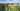The average SAT score of 1020 breaks down into:

• SAT math: 530

The average ACT score for Arkansas State University is 24 and their acceptance rate is 70.2%.

## North Arkansas College SAT scores

The average SAT score for North Arkansas College is 1040.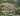The average SAT score of 1040 breaks down into:

• SAT math: 520

The average ACT score for North Arkansas College is 20 and their acceptance rate is 100%.

## Henderson State University SAT scores

The average SAT score for Henderson State University is 1060.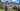The average SAT score of 1060 breaks down into:

• SAT math: 530

The average ACT score for Henderson State University is 22 and their acceptance rate is 90.5%.

## University of Arkansas at Little Rock SAT scores

The average SAT score for University of Arkansas at Little Rock is 1060.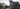The average SAT score of 1060 breaks down into:

• SAT math: 540

The average ACT score for University of Arkansas at Little Rock is 23 and their acceptance rate is 59%.

## University of the Ozarks SAT scores

The average SAT score for University of the Ozarks is 1070.The average SAT score of 1070 breaks down into:

• SAT math: 530

The average ACT score for University of the Ozarks is 23 and their acceptance rate is 97%.

## University of Central Arkansas SAT scores

The average SAT score for University of Central Arkansas is 1070.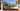The average SAT score of 1070 breaks down into:

• SAT math: 540

The average ACT score for University of Central Arkansas is 24 and their acceptance rate is 91.1%.

## Arkansas Tech University SAT scores

The average SAT score for Arkansas Tech University is 1100.The average SAT score of 1100 breaks down into:

• SAT math: 560

The average ACT score for Arkansas Tech University is 22 and their acceptance rate is 90.2%.

## Southern Arkansas University Magnolia SAT scores

The average SAT score for Southern Arkansas University Magnolia is 1103.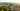The average SAT score of 1103 breaks down into:

• SAT math: 540